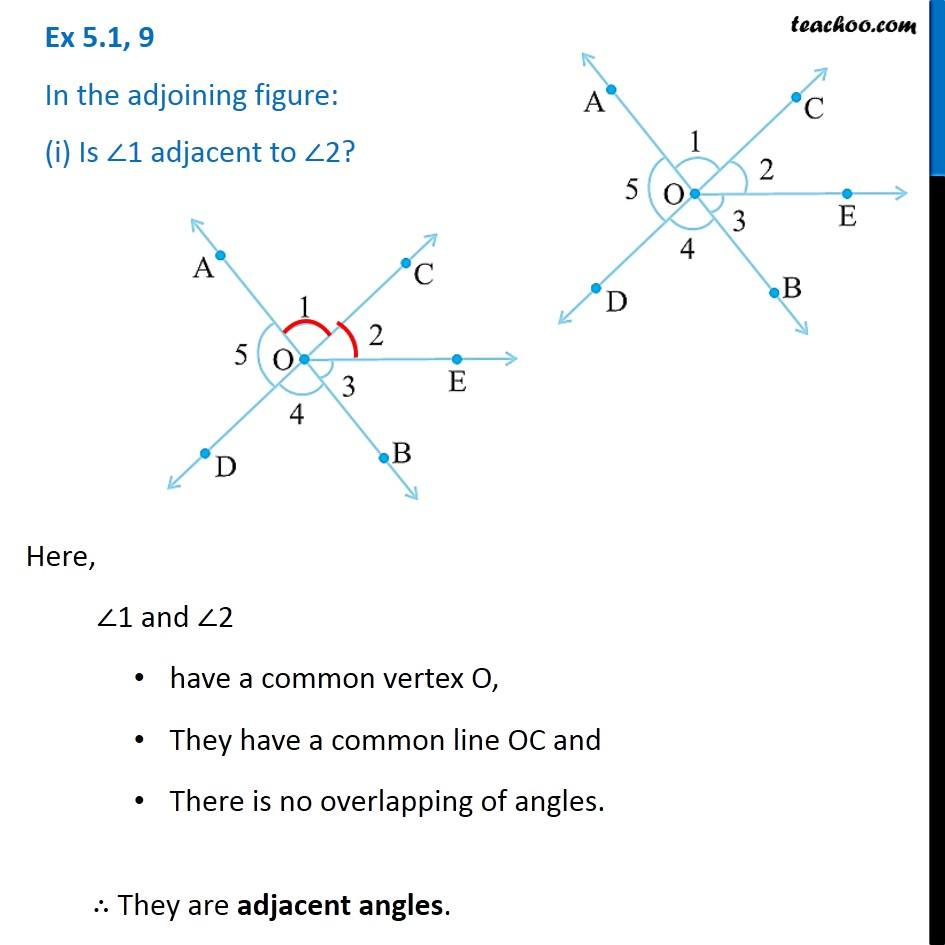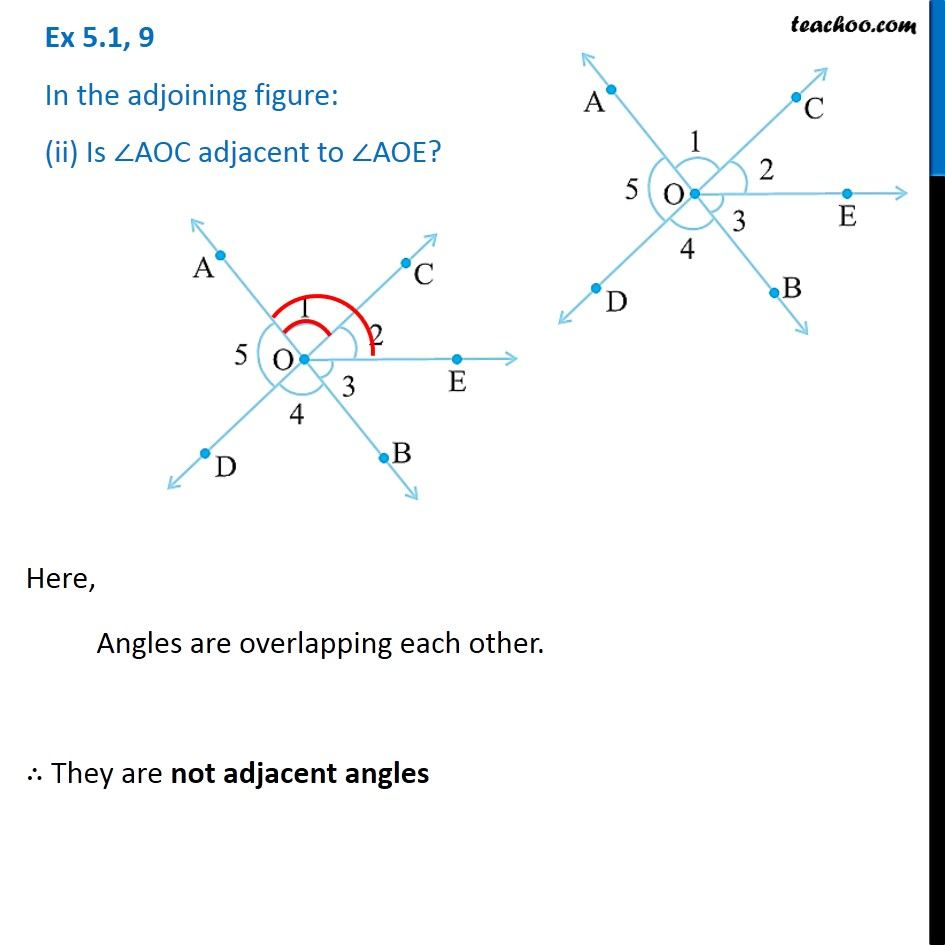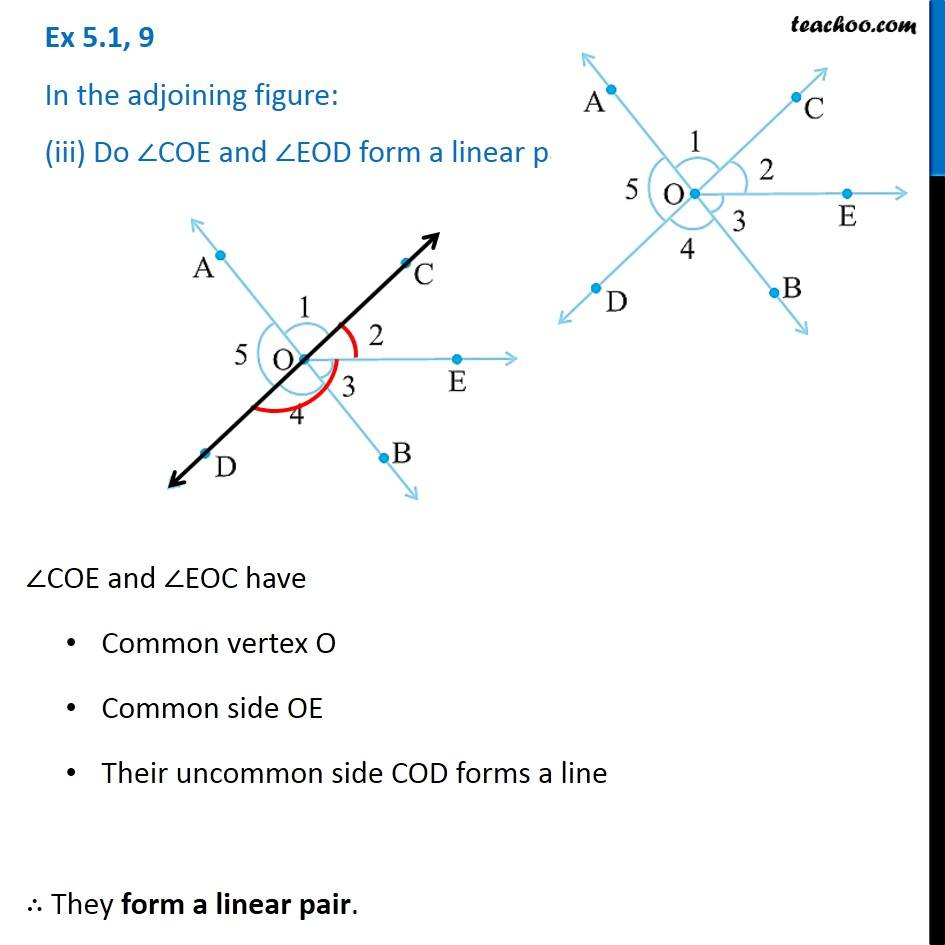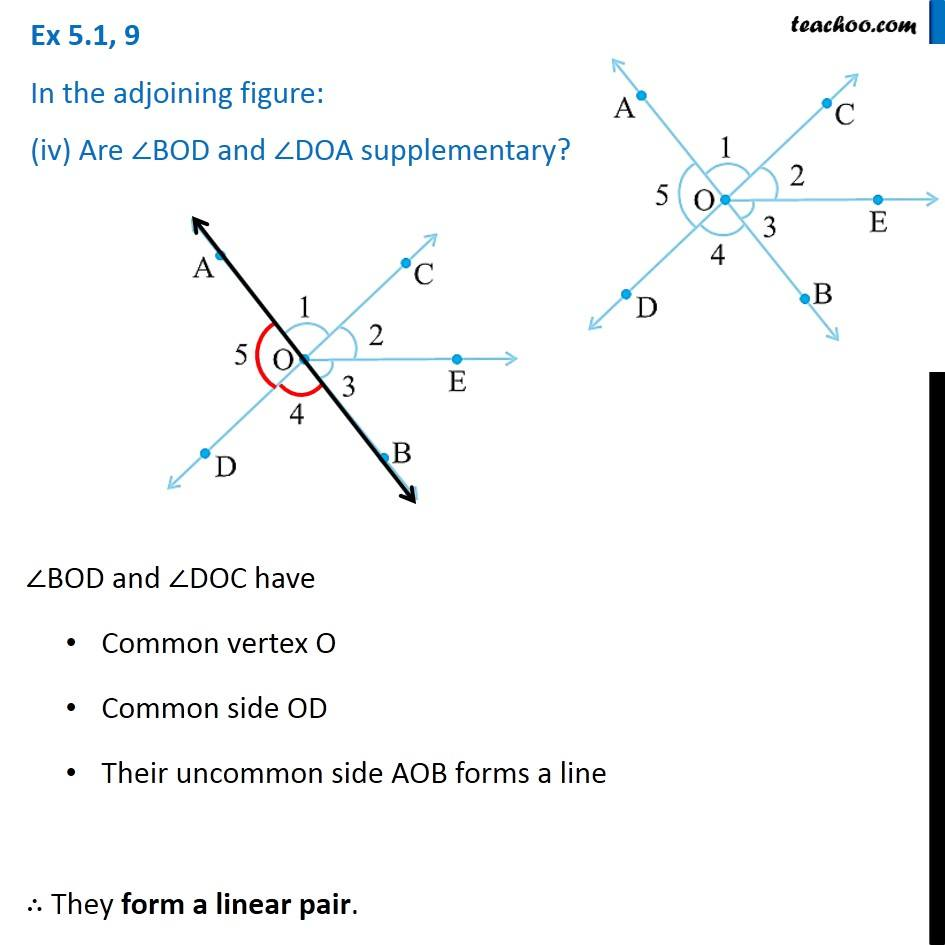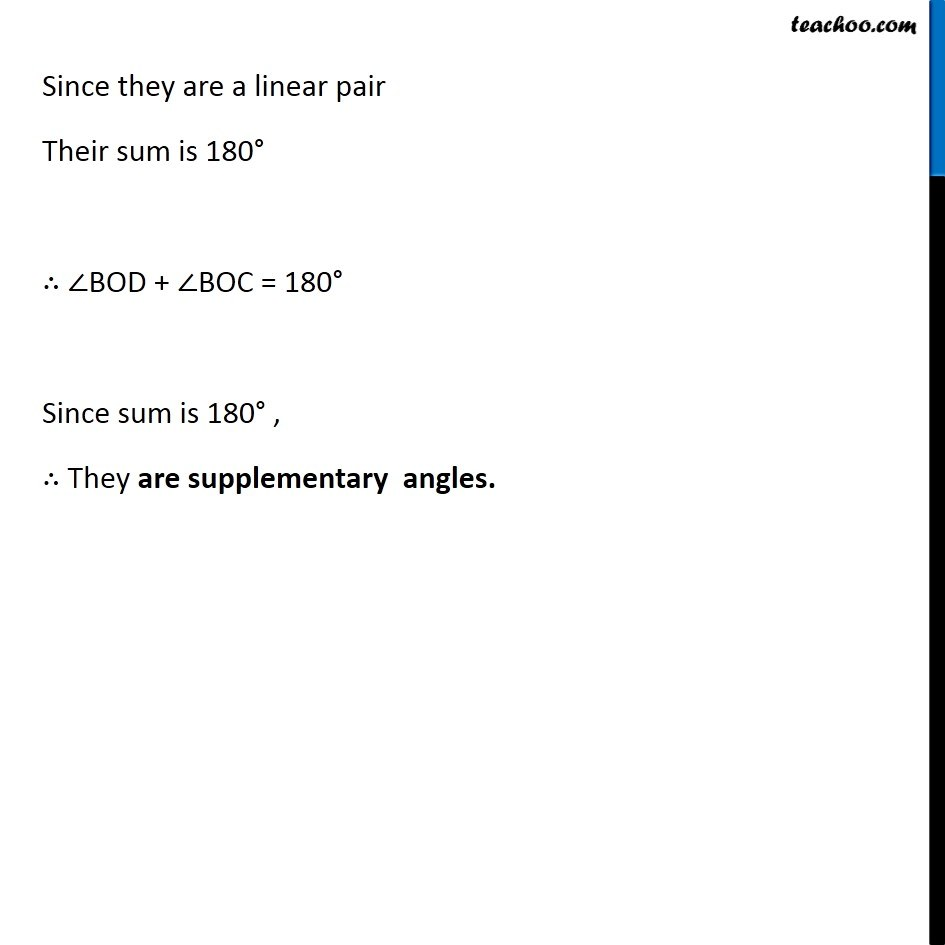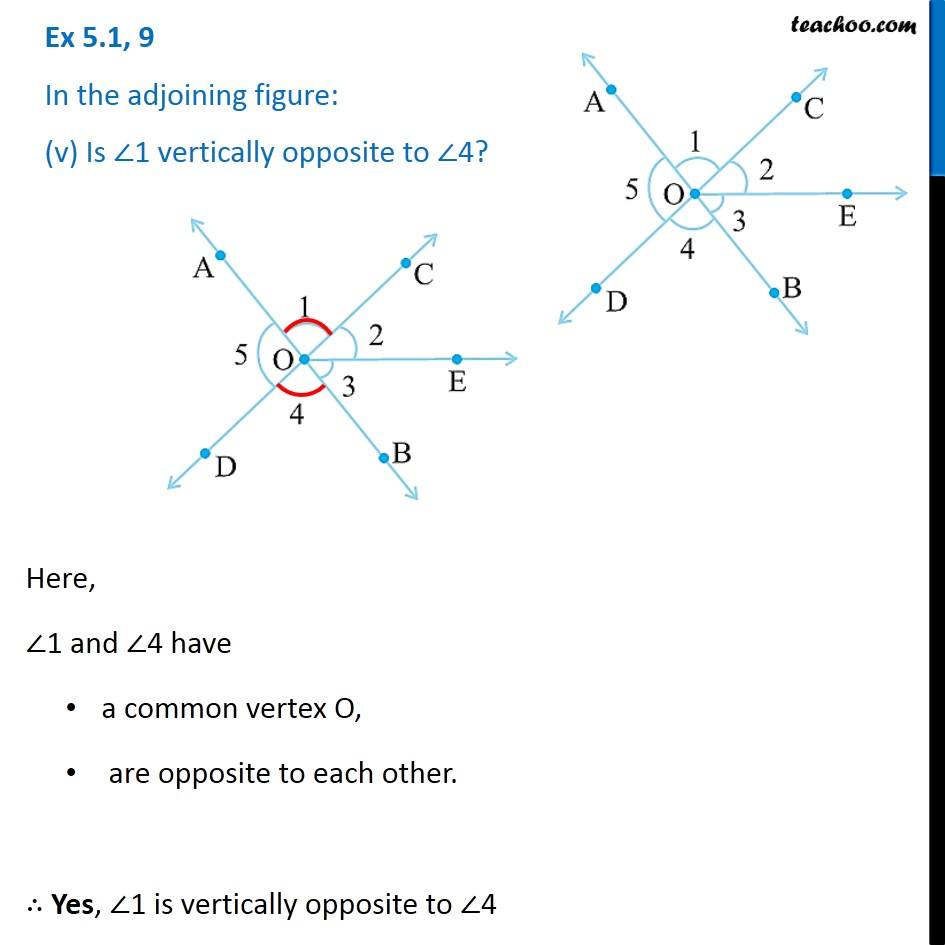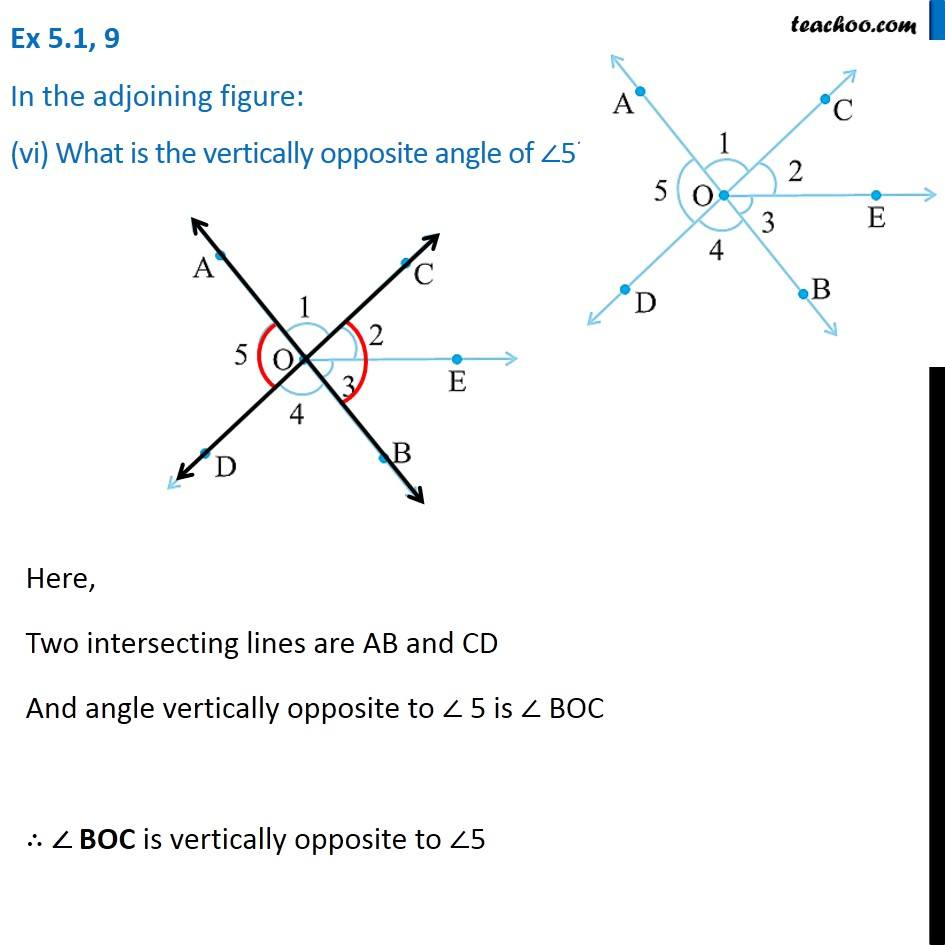1. Chapter 5 Class 7 Lines and Angles
2. Serial order wise
3. Ex 5.1

Transcript

Ex 5.1, 9 In the adjoining figure: (i) Is ∠1 adjacent to ∠2? Here, ∠1 and ∠2 have a common vertex O, They have a common line OC and There is no overlapping of angles. ∴ They are adjacent angles. Ex 5.1, 9 In the adjoining figure: (ii) Is ∠AOC adjacent to ∠AOE?Here, Angles are overlapping each other. ∴ They are not adjacent angles Ex 5.1, 9 In the adjoining figure: (iii) Do ∠COE and ∠EOD form a linear pairCOE and ∠EOC have Common vertex O Common side OE Their uncommon side COD forms a line ∴ They form a linear pair. Ex 5.1, 9 In the adjoining figure: (iv) Are ∠BOD and ∠DOA supplementary? ∠BOD and ∠DOC have Common vertex O Common side OD Their uncommon side AOB forms a line ∴ They form a linear pair. ∠BOD and ∠DOC have Common vertex O Common side OD Their uncommon side AOB forms a line ∴ They form a linear pair. Ex 5.1, 9 In the adjoining figure: (v) Is ∠1 vertically opposite to ∠4? Here, ∠1 and ∠4 have a common vertex O, are opposite to each other. ∴ Yes, ∠1 is vertically opposite to ∠4 Ex 5.1, 9 In the adjoining figure: (vi) What is the vertically opposite angle of ∠5? Here, Two intersecting lines are AB and CD And angle vertically opposite to ∠ 5 is ∠ BOC ∴ ∠ BOC is vertically opposite to ∠5

Ex 5.1

Chapter 5 Class 7 Lines and Angles
Serial order wise